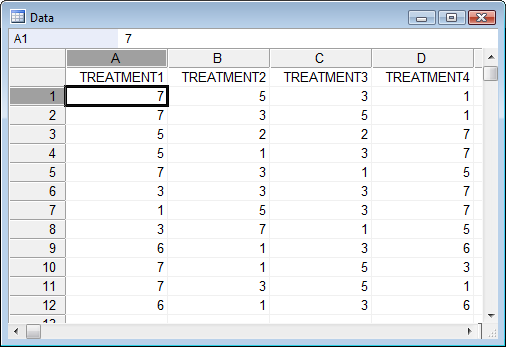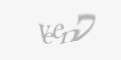Most often, repeated measures ANOVA test is the first choice among the researchers for determining the difference between 3 or more variables. However, if assumptions such as normal distribution isn’t met, an alternative test known as Friedman test is used.

Friedman test, an extended version of one-way ANOVA with repeated measures, is a non-parametric test used to determine the difference between 3 or more matched or paired groups. Basic ANOVA test has the assumptions of a normal distribution with their corresponding variances, but Friedman test eliminates these assumptions of normal distribution. The method incorporates ranking for each block together afterwards analyzing the values of ranks in each column. The Friedman test is primarily a 2-way ANOVA used for the data that is Non - parametric.

In Friedman test one variable serves as a treatment/group variable and another as a blocking variable. Here, the dependent variable must be continuous (but not normally distributed) and independent variable must be categorical (time/condition)

Like any other statistical test, this test too consists of a few assumptions including:

• The samples should not be normally distributed

• There is one group that is measured on three or more occasions

• The dependent variable must be measured at an ordinal or continuous level

• A group is a random sample from the population

Prior to conducting this test, a researcher must set up hypothesis such as:

• Null hypothesis - It states that the medians of values of each group are equal. Simply put, the treatments have no effect

• Alternative hypothesis - The medians of values of each group are not equal indicating that there is a difference between the treatments

Today, although several tools such as SPSS, SAS, etc. are used to perform Friedman test, the most tool among the researchers is R language.

So, how do you conduct this test in R?

In order to conduct this test in R, import the file into R and refer to the variables directly within the data set. This is followed by analysing the data using the command “Friedman.test.” Create a matrix or table, fill the data and run the test using the “Friedman test ()” command.Upon completion of the analysis, the next step is to interpret the results. I.e. to check if the test is statistically significant or not. To accomplish this, compare the P value with the significance level.

However, before interpreting the results, it should be noted that the Friedman test ranks values in each row. As a result, the test is not affected by sources of variability that equally affect all values in a row. Typically, a significance level of 0.05 works well. We check the value at 5 % significance level.

• P-value ≤ significance level : If the p-value less than significance level, then we can reject the idea that the difference between the columns are the result of random sampling, concluding that at least one column differs from another.

•  P-value > significance level : If the P value is greater than the significance level, then the data doesn’t provide you with significant evidence to conclude that overall medians differ. However, this isn’t similar to stating that all medians are similar.

Although the outcome of Friedman tells you if the groups are significantly different from each other, they do not tell you which groups differ from each other. This is when post hoc analysis for the Friedman’s test comes into the picture.

The primary goal here is to investigate which all pairs of groups are significantly different from each other. If you have N groups, to check all of their pairs, you will have to perform n^2 comparisons, therefore the need of correcting multiple comparisons arise.

The initial step in a post hoc analysis in R to find out which groups are responsible for the rejection of the null hypothesis. For simple ANOVA test, there exists a readily available package that can directly calculate post hoc analysis -TukeyHSD.

This is followed by understanding the outputs from the test run. In the case of simple ANOVA, a box plot would be sufficient, but in the case of repeated measure test, a boxplot approach can be misleading. Therefore you can consider using two plots: (a) one for parallel coordinates (b) other boxplots of the differences between all pairs.

Glimpse at an example for Friedman test

Consider an experiment where 6 persons (block) received 6 different diuretics (groups) that were A to F. Here the response determines the concentration of Na in human urine and the observations were recorded after each treatment.

> require(PMCMR) [library used to perform Friedman test]

> r <- matrix(c(

+ 3.88, 5.44, 8.96,8 .25, 4.91, 12.33, 28.58, 31.14, 16.92,

+ 24.19, 26.84, 10.91, 25.24, 39.52, 25.45, 16.85, 20.45,

+ 28.67, 4.44, 7.94, 4.04, 4.4, 4.23, 4.36, 29.41, 37.72,

+ 39.92, 28.23, 28.35, 12, 38.87, 35.12, 39.15, 28.06, 38.23,

+ 26.65),

Nrow = 6,

Ncol = 6,

+ dimnames = list (1:,c("a”,"b","g","h","i","j")))

> print (r)

a b g h i j 1 3.88 5.4 48.96 8 .25 4.91 12.33 28.58 31.14 16.92,

24.19 26.8 10.91 25.2 39.5 25.45 16.85 20.45

28.67 4.4 47.94 4.04 4.4 4.23 4.3629.41 37.72

39.9228.23 28.35 12 38.87 35.12 39.15 28.06 38.23

26.65

STS > friedman .test(y)

Friedman chi-squared (χ 2) = 23.333,

Degree of freedom = 5 , p-value = 0.000287

Result -  using friedman test

χ 2 (5) = 23.3- chi square test

p < 0.01

Note:

A different post hoc tests can be performed by using the command posthoc.friedman.conover.test in the PMCMR package.